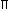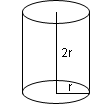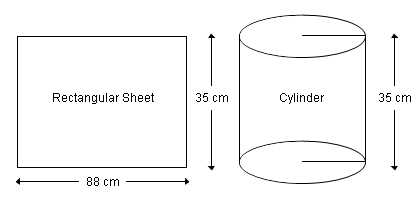1.
The radius of a sphere is r units. Each of the radius of the base and the height of a right circular cylinder is also r units. What is the ratio of the volume of the sphere to that of the cylinder?
• A.
2 : 3
• C.
3 : 2
• B.
3 : 4
• D.
4 : 3
• Answer & Explanation
• Report
Explanation :
Volume of sphere =
 4 3r3
and Volume of cylinder =r2h
=r3

Required ratio =
 4 3r3
:r3
=
 4 3
: 1 = 4 : 3
Report
 Name Email
2.
The total surface area of a sphere is S1 and the curved surface area of a cylinder enclosing it is S2. Then S1 : S2 will be equal to
• A.
1 : 3
• C.
2 : 1
• B.
1 : 2
• D.
1 : 1
• Answer & Explanation
• Report
Explanation :
Let r be the radius of the sphere
 Surface area of the sphere = S1 = 4r2Since the cylinder encloses the sphere, height of cylinder = 2r and radius = r
Let S2 be the surface area of the cylinder
S2 = 2rh = 2r(2r) = 4r2
 S1 S2
=
 4r
 4r
= 1 : 1
Report
 Name Email
3.
A rectangular room of size 5m (length) x 4m (width) x 3m (height) is to be painted. If the unit cost of painting is Rs. 10 per sq.m., what is the total cost of painting?
• A.
Rs. 640
• C.
Rs. 740
• B.
Rs. 800
• D.
Rs. 940
• Answer & Explanation
• Report
Explanation :
Area of 4 walls = 2h (l + b)
The area to be painted includes the 4 walls and the top ceiling.
Total area to be painted = 2h (l + b) + lb = 2 x 3 (5 + 4) + 5 x 4
 = 54 + 20 = 74 sq.m
Total cost of painting = 74 x 10 = Rs. 740
Report
 Name Email
4.
A rectangular sheet of size 88cm x 35 cm is bent to form a cylindrical shape with height 35 cm. What is the area of the base of the cylindrical shape?
• A.
600 sq.cm
• C.
88 sq.cm
• B.
676 sq.cm
• D.
616 sq.cm
• Answer & Explanation
• Report
Explanation :The circumference of the circular region = 88 cm
 2r = 88 r = 88 x
 1 2
x
 7 22
= 14 cm
 Area of the base =r2
 22 7
x 14 x 14 = 616 cm2
Report
 Name Email
5.
Volume of a right circular cylinder and that of a sphere are equal. If the radius of the base of the cylinder is the same as that of the sphere, which of the following is true as regards the height of the cylinder?
• A.
It is 4/3 rd of its radius
• C.
It is equal to its diameter
• B.
It is equal to its radius
• D.
It is 2/3 rd of its radius
• Answer & Explanation
• Report
Explanation :
Volume of cylinder = Volume of sphere
Both the radius are also equalr2h =
 4 3r3
h =
 4 3
r
Height is
 4 3